SSC CGL Tier 1 (19 October) Shift 1 Past Year 2014 Paper

# SSC CGL Tier 1 (19 October) Shift 1 Past Year 2014 Paper - SSC CGL

Test Description

## 201 Questions MCQ Test SSC CGL Tier 1 Mock Test Series - SSC CGL Tier 1 (19 October) Shift 1 Past Year 2014 Paper

SSC CGL Tier 1 (19 October) Shift 1 Past Year 2014 Paper for SSC CGL 2023 is part of SSC CGL Tier 1 Mock Test Series preparation. The SSC CGL Tier 1 (19 October) Shift 1 Past Year 2014 Paper questions and answers have been prepared according to the SSC CGL exam syllabus.The SSC CGL Tier 1 (19 October) Shift 1 Past Year 2014 Paper MCQs are made for SSC CGL 2023 Exam. Find important definitions, questions, notes, meanings, examples, exercises, MCQs and online tests for SSC CGL Tier 1 (19 October) Shift 1 Past Year 2014 Paper below.
Solutions of SSC CGL Tier 1 (19 October) Shift 1 Past Year 2014 Paper questions in English are available as part of our SSC CGL Tier 1 Mock Test Series for SSC CGL & SSC CGL Tier 1 (19 October) Shift 1 Past Year 2014 Paper solutions in Hindi for SSC CGL Tier 1 Mock Test Series course. Download more important topics, notes, lectures and mock test series for SSC CGL Exam by signing up for free. Attempt SSC CGL Tier 1 (19 October) Shift 1 Past Year 2014 Paper | 201 questions in 300 minutes | Mock test for SSC CGL preparation | Free important questions MCQ to study SSC CGL Tier 1 Mock Test Series for SSC CGL Exam | Download free PDF with solutions
 1 Crore+ students have signed up on EduRev. Have you?
SSC CGL Tier 1 (19 October) Shift 1 Past Year 2014 Paper - Question 1

### Which of the following interchange of signs would make the equation correct ? 8 × 6 +2 = 22

Detailed Solution for SSC CGL Tier 1 (19 October) Shift 1 Past Year 2014 Paper - Question 1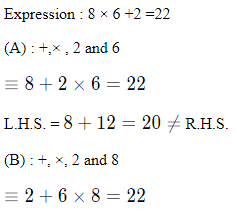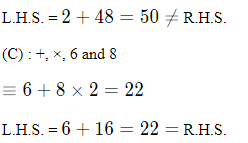SSC CGL Tier 1 (19 October) Shift 1 Past Year 2014 Paper - Question 2

### If ‘-‘ stands for addition, ‘+’ stands for subtraction, ‘÷’ stands for multiplication and ‘×’ stands for division, then which one of the following equation is correct ?

Detailed Solution for SSC CGL Tier 1 (19 October) Shift 1 Past Year 2014 Paper - Question 2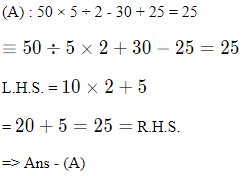SSC CGL Tier 1 (19 October) Shift 1 Past Year 2014 Paper - Question 3

### In each of the following questions, some equations are solved on the basis of certain system. Find out the correct answer for the unsolved equation on that basis. 2 × 4 × 6 = 4; 9 x 3 x 7 = 13; 4 × 7 × 6=3; 9 × 7 × 8 = ?

Detailed Solution for SSC CGL Tier 1 (19 October) Shift 1 Past Year 2014 Paper - Question 3

The pattern followed is that all the numbers are added and twice of the middle number is subtracted from the sum to obtain final result.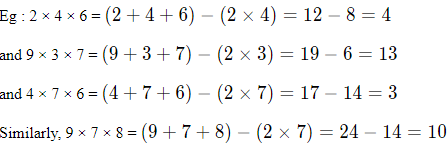SSC CGL Tier 1 (19 October) Shift 1 Past Year 2014 Paper - Question 4

3 × 5 × 7 × 2 = 24, 2 × 4 × 6 × 8 = 22, 4 × 4 × 8 × 9 = ?

Detailed Solution for SSC CGL Tier 1 (19 October) Shift 1 Past Year 2014 Paper - Question 4

The product of the first two numbers is added to the sum of last two numbers.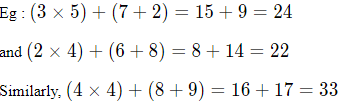SSC CGL Tier 1 (19 October) Shift 1 Past Year 2014 Paper - Question 5

In each of the following questions, select the missing number from the given responses: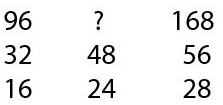Detailed Solution for SSC CGL Tier 1 (19 October) Shift 1 Past Year 2014 Paper - Question 5let the missing term be y

here , in 1st column ,

16 x 2 = 32

32 x 3 = 96

in 3rd column ,

28 x 2 = 56

56 x 3 = 168

so in 2nd column the same pattern is going to get followed

24 x 2 = 48

48 x 3 = 144

and hence y = 144

SSC CGL Tier 1 (19 October) Shift 1 Past Year 2014 Paper - Question 6

In each of the following questions, select the missing number from the given responses: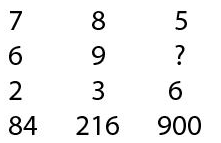Detailed Solution for SSC CGL Tier 1 (19 October) Shift 1 Past Year 2014 Paper - Question 6let the missing term be y

here in 1st column we can see that 84 = 7 x 6 x 2

in 2nd column we can see that 216 = 8 x 9 x 3

so in 3rd column the same thing is going to get followed ,

and hence 900 = 5 x y x 6

y = 30

SSC CGL Tier 1 (19 October) Shift 1 Past Year 2014 Paper - Question 7

In each of the following questions, select the missing number from the given responses: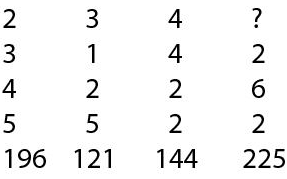Detailed Solution for SSC CGL Tier 1 (19 October) Shift 1 Past Year 2014 Paper - Question 7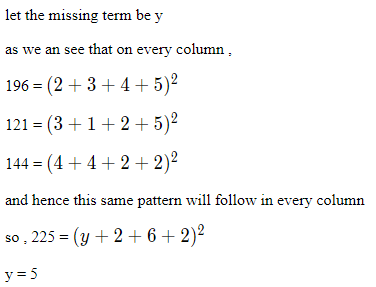SSC CGL Tier 1 (19 October) Shift 1 Past Year 2014 Paper - Question 8

In each of the following questions, select the related word/letters/numbers from the given alternatives.

SOCIAL : OCIALS : : DRIVEN : ?

Detailed Solution for SSC CGL Tier 1 (19 October) Shift 1 Past Year 2014 Paper - Question 8

we are given that SOCIAL : OCIALS : : DRIVEN : ?

in SOCIAL : OCIALS , we can see that first alphabet is shifted to last

and hence the same thing will be done in 2nd case

and hence DRIVEN : RIVEND

SSC CGL Tier 1 (19 October) Shift 1 Past Year 2014 Paper - Question 9

In each of the following questions, select the related word/letters/numbers from the given alternatives.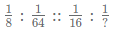Detailed Solution for SSC CGL Tier 1 (19 October) Shift 1 Past Year 2014 Paper - Question 9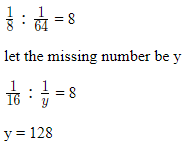SSC CGL Tier 1 (19 October) Shift 1 Past Year 2014 Paper - Question 10

In each of the following questions, select the related word/letters/numbers from the given alternatives.

29 : 87 : : 79 : ?

Detailed Solution for SSC CGL Tier 1 (19 October) Shift 1 Past Year 2014 Paper - Question 10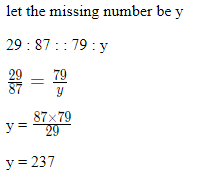SSC CGL Tier 1 (19 October) Shift 1 Past Year 2014 Paper - Question 11

In each of the following questions, select the related word/letters/numbers from the given alternatives.

Embarrassed : Humiliated :: Frightened : ?

Detailed Solution for SSC CGL Tier 1 (19 October) Shift 1 Past Year 2014 Paper - Question 11

Embarrassed and humiliated are synonyms, thus the word with similar meaning as frightened is terrified.

SSC CGL Tier 1 (19 October) Shift 1 Past Year 2014 Paper - Question 12

In each of the following questions, select the related word/letters/numbers from the given alternatives.

Macabre : Lovely : : Baneful : ?

Detailed Solution for SSC CGL Tier 1 (19 October) Shift 1 Past Year 2014 Paper - Question 12

Macabre and lovely are antonyms, thus the word with opposite in meaning as baneful (harmful) is unharmful.

SSC CGL Tier 1 (19 October) Shift 1 Past Year 2014 Paper - Question 13

In each of the following questions, select the related word/letters/numbers from the given alternatives.

AYRRJC : CATTLE : : NCPDCAR : ?

Detailed Solution for SSC CGL Tier 1 (19 October) Shift 1 Past Year 2014 Paper - Question 13

Expression = AYRRJC : CATTLE

The pattern followed is :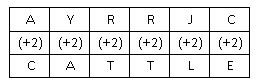Similarly, for NCPDCAR :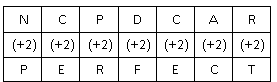SSC CGL Tier 1 (19 October) Shift 1 Past Year 2014 Paper - Question 14

In each of following questions, find the odd word/number/letters/number pair from the given alternatives:

Detailed Solution for SSC CGL Tier 1 (19 October) Shift 1 Past Year 2014 Paper - Question 14

Square, trapezium and parallelogram are 2 dimensional figure, hence cylinder which is a 3-D figure is the odd one.

SSC CGL Tier 1 (19 October) Shift 1 Past Year 2014 Paper - Question 15

In each of following questions, find the odd word/number/letters/number pair from the given alternatives:

Detailed Solution for SSC CGL Tier 1 (19 October) Shift 1 Past Year 2014 Paper - Question 15

All except a submarine sail on the water, while a submarine is submerged into it, hence it is the odd one out.

SSC CGL Tier 1 (19 October) Shift 1 Past Year 2014 Paper - Question 16

In each of following questions, find the odd word/number/letters/number pair from the given alternatives:

Detailed Solution for SSC CGL Tier 1 (19 October) Shift 1 Past Year 2014 Paper - Question 16

Malayalam is a language of Kerala, hence it is the odd one.

SSC CGL Tier 1 (19 October) Shift 1 Past Year 2014 Paper - Question 17

In each of following questions, find the odd word/number/letters/number pair from the given alternatives:

Detailed Solution for SSC CGL Tier 1 (19 October) Shift 1 Past Year 2014 Paper - Question 17

(A) : M (-4 letters) = I (-2 letters) = G (-2 letters) = E

(B) : X (-4 letters) = T (-3 letters) = Q (-2 letters) = O

(C) : R (-4 letters) = N (-3 letters) = K (-2 letters) = I

(D) : H (-4 letters) = D (-3 letters) = A (-2 letters) = Y

SSC CGL Tier 1 (19 October) Shift 1 Past Year 2014 Paper - Question 18

In each of following questions, find the odd word/number/letters/number pair from the given alternatives:

Detailed Solution for SSC CGL Tier 1 (19 October) Shift 1 Past Year 2014 Paper - Question 18

All the numbers except 42 are completely divisible by the second number, hence 42 : 4 is the odd one out.

SSC CGL Tier 1 (19 October) Shift 1 Past Year 2014 Paper - Question 19

In each of following questions, find the odd word/number/letters/number pair from the given alternatives:

Detailed Solution for SSC CGL Tier 1 (19 October) Shift 1 Past Year 2014 Paper - Question 19

Among all the given options, only year 2012 is a leap year, hence it is the odd one.

SSC CGL Tier 1 (19 October) Shift 1 Past Year 2014 Paper - Question 20

Arrange the following words as per order in the dictionary :

I. Euphrasy
II. Eupepsy
III. Euphonic
IV. Eugenic
V. Euphony

Detailed Solution for SSC CGL Tier 1 (19 October) Shift 1 Past Year 2014 Paper - Question 20

As per order in the dictionary :

= Eugenic -> Eupepsy -> Euphonic -> Euphony -> Euphrasy

≡ IV, II, III, V, I

SSC CGL Tier 1 (19 October) Shift 1 Past Year 2014 Paper - Question 21

Which one of the given responses would be a meaningful order of the following ?

I. Absorption
II. Digestion
III. Nutrition
IV. Excretion

Detailed Solution for SSC CGL Tier 1 (19 October) Shift 1 Past Year 2014 Paper - Question 21

The process that happens internally in a body follows the order :

= Nutrition -> Digestion -> Absorption -> Excretion

≡ III, II, I, IV

SSC CGL Tier 1 (19 October) Shift 1 Past Year 2014 Paper - Question 22

In each of the following questions, a series is given, with one term missing. Choose the correct alternative from the given ones that will complete the series

0, ? , 8 27, 64, 125

Detailed Solution for SSC CGL Tier 1 (19 October) Shift 1 Past Year 2014 Paper - Question 22

here the given pattern is 0, ? , 8 27, 64, 125

let the missing term be y

as we can see that every pateern is a cube of consecutive whole number starting from 0

0 = 03

8 = 23

64 = 43

125 = 53

so the missing term is , y = 13 = 1

SSC CGL Tier 1 (19 October) Shift 1 Past Year 2014 Paper - Question 23

In each of the following questions, a series is given, with one term missing. Choose the correct alternative from the given ones that will complete the series

BMX, DNW, FOV, ? .

Detailed Solution for SSC CGL Tier 1 (19 October) Shift 1 Past Year 2014 Paper - Question 23

here the given series is BMX, DNW, FOV, ?

as we can see that 1st alphabet of every term in series is alternative element starting from B

2nd alphabet if every term series is consecutive alphabets starting from M and 3rd alphabet in every term in the series is retracing back every consecutive alphabet starting from X

and hence the missing pattern is HPU

SSC CGL Tier 1 (19 October) Shift 1 Past Year 2014 Paper - Question 24

In each of the following questions, a series is given, with one term missing. Choose the correct alternative from the given ones that will complete the series

655, 637, 622, 610, 601, ?

Detailed Solution for SSC CGL Tier 1 (19 October) Shift 1 Past Year 2014 Paper - Question 24

Let the missing number be x.

The difference between every two consecutive numbers is forming a pattern.

655-637 = 18

637-622 = 15

622-610 = 12

610-601 = 9

As the difference is getting reduced by 3 every time . So 601-x = 6 which implies that the missing number ,x =595

SSC CGL Tier 1 (19 October) Shift 1 Past Year 2014 Paper - Question 25

In each of the following questions, a series is given, with one term missing. Choose the correct alternative from the given ones that will complete the series

1, 5, 25, 125, ? , ? , ?

Detailed Solution for SSC CGL Tier 1 (19 October) Shift 1 Past Year 2014 Paper - Question 25

we have been give a series as 1, 5, 25, 125, ? , ? , ?

here we can see that every term is a power of 5

1 = 50

5 = 51

25 = 52

125 = 53

so the next term will also be power of 5 and the terms are 54, 55 , 56 i,e 625, 3125, 15625

SSC CGL Tier 1 (19 October) Shift 1 Past Year 2014 Paper - Question 26

In a class composed of x girls y boys what part of the class is composed of girls ?

Detailed Solution for SSC CGL Tier 1 (19 October) Shift 1 Past Year 2014 Paper - Question 26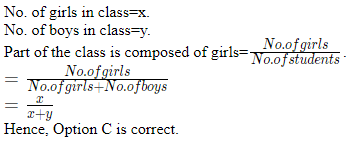SSC CGL Tier 1 (19 October) Shift 1 Past Year 2014 Paper - Question 27

If a number is greater than 5 but less than 9 and greater than 7 but less than 11, the number is

Detailed Solution for SSC CGL Tier 1 (19 October) Shift 1 Past Year 2014 Paper - Question 27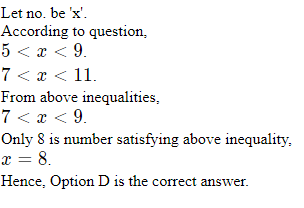SSC CGL Tier 1 (19 October) Shift 1 Past Year 2014 Paper - Question 28

In a row, 25 trees are planted at equal distance from each other. The distance between 1st and 25th tree is 30 m. What is the distance between 3rd and 15th tree?

Detailed Solution for SSC CGL Tier 1 (19 October) Shift 1 Past Year 2014 Paper - Question 28

Let distance between two consecutive trees be 'x' m.
Distance between 1st and 25th tree = 24x.
According to question, 24x=30
x=1.25
Distance between 3rd and 15th tree=12x.
=12×1.25.
=15 m.
Hence, Option B is correct.

SSC CGL Tier 1 (19 October) Shift 1 Past Year 2014 Paper - Question 29

In each of the following questions, from the given alternative words, select the word which can be formed using the letters of the given word :

INSTITUTIONALISE

Detailed Solution for SSC CGL Tier 1 (19 October) Shift 1 Past Year 2014 Paper - Question 29

The word INSTITUTIONALISE does not contain any 'R', hence the word 'Nutrition' cannot be formed.

SSC CGL Tier 1 (19 October) Shift 1 Past Year 2014 Paper - Question 30

In each of the following questions, from the given alternative words, select the word which can be formed using the letters of the given word :

STIMULATION

Detailed Solution for SSC CGL Tier 1 (19 October) Shift 1 Past Year 2014 Paper - Question 30

The word STIMULATION does not contain 2 N's, or 2 O's or any H, hence the last three words cannot be formed.

Only word that can be formed : Station

SSC CGL Tier 1 (19 October) Shift 1 Past Year 2014 Paper - Question 31

In a certain code, TRIPPLE is written as SQHOOKD. How is DISPOSE written in that code?

Detailed Solution for SSC CGL Tier 1 (19 October) Shift 1 Past Year 2014 Paper - Question 31

TRIPPLE is written as SQHOOKD

The pattern followed is :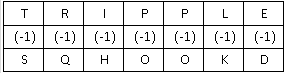Similarly, for DISPOSE :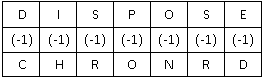SSC CGL Tier 1 (19 October) Shift 1 Past Year 2014 Paper - Question 32

Which letter in the word ‘Vertex’ should be changed to mean spiral movement ?

Detailed Solution for SSC CGL Tier 1 (19 October) Shift 1 Past Year 2014 Paper - Question 32

Vortex means spiral movement. Thus, 2nd letter in the word 'Vertex', i.e., 'e' is changed to 'o'.

SSC CGL Tier 1 (19 October) Shift 1 Past Year 2014 Paper - Question 33

If TRANSFER is coded as RTNAFSRE, then how ELEPHANT be coded in that code language ?

Detailed Solution for SSC CGL Tier 1 (19 October) Shift 1 Past Year 2014 Paper - Question 33

Given : TRANSFER is coded as RTNAFSRE

The pattern is that in groups of 2 letters (from either side), both letters are swapped.

Eg = TR : RT , AN : NA , SF : FS , ER : RE

Similarly, for EL EP HA NT : LE PE AH TN

Thus, ELEPHANT is written as LEPEAHTN

SSC CGL Tier 1 (19 October) Shift 1 Past Year 2014 Paper - Question 34

If MONKO is coded as 57637, then how KLJMN be coded in the same code ?

Detailed Solution for SSC CGL Tier 1 (19 October) Shift 1 Past Year 2014 Paper - Question 34

Given : MONKO is coded as 57637

Thus, I = 1 , J = 2 , K = 3 , L = 4 , M = 5 and so on.

Similarly, KLJMN = 34256

SSC CGL Tier 1 (19 October) Shift 1 Past Year 2014 Paper - Question 35

Statement is given followed by two inferences I and II. You have to consider the statement to be true even if it seems to be at variance from commonly known facts. You have to decide which of the given inferences, if any. follow from the given statement.

Statement :

“Electric supply in Anand Colony will be cut tomorrow after 12 O’clock for three hours because repairing work will be carried out.”

Inferences :
I. Residents of Anand Colony may use their electrical appliances before 12 O’clock tomorrow.
II. Residents of Anand Colony need training for using electricity economically.

Detailed Solution for SSC CGL Tier 1 (19 October) Shift 1 Past Year 2014 Paper - Question 35

The given statement is a notice for the residents of Anand Colony to inform them about the electricity cut so that they use their electric appliances before 12 o’clock. The statement has no reference to whether or not the residents are using electricity economically.

Hence, only inference I follows.

SSC CGL Tier 1 (19 October) Shift 1 Past Year 2014 Paper - Question 36

Anand travels 10 kms from his home to the east to reach his school. Then he travels 5 kms to the south to reach his father’s shop, after school. He then travels 10 kms to the west to help his uncle. How far and in which direction is he from his home ?

Detailed Solution for SSC CGL Tier 1 (19 October) Shift 1 Past Year 2014 Paper - Question 36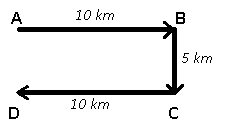Let Anand started from point A and travelled east for 10 km to reach his school at B, then travelled south for 5 km to reach his father's shop at C, finally travelled west for 10 km to stop at point D.

\therefore∴ Anand is 5 km to the south of his home.

SSC CGL Tier 1 (19 October) Shift 1 Past Year 2014 Paper - Question 37

Four villages A, B, C and D lie in a straight line. D is 10 kms from B. A is exactly Between D and C and C from B is 2 kms more than it is from D. How far is C from B ?

Detailed Solution for SSC CGL Tier 1 (19 October) Shift 1 Past Year 2014 Paper - Question 37

Distance between D and B = 10 km

C from B is 2 km more than it is from D, that means DC = 4 km and CB = 6 km

A is exactly between D and C i.e. AD = 2 km and AC = 2 km

Following figure represents all the above conclusions.Hence CB = 6 km

SSC CGL Tier 1 (19 October) Shift 1 Past Year 2014 Paper - Question 38

Select the alternative inference which is most appropriate. “All professors are learned; learned people are always gentle.”Inference : All professors are gentle persons.

Detailed Solution for SSC CGL Tier 1 (19 October) Shift 1 Past Year 2014 Paper - Question 38

The venn diagram for above statements is :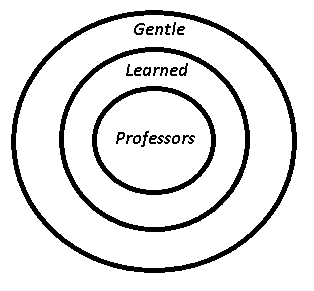Inference : All professors are gentle persons = true

SSC CGL Tier 1 (19 October) Shift 1 Past Year 2014 Paper - Question 39

In each of the following questions, among four answer figures whose cut pieces can form the given question figure?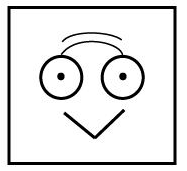Detailed Solution for SSC CGL Tier 1 (19 October) Shift 1 Past Year 2014 Paper - Question 39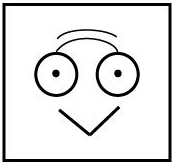By removing dots from circles and placing them above the straight lines, we can get figure in Option D.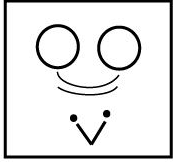Hence, Option D is correct.

SSC CGL Tier 1 (19 October) Shift 1 Past Year 2014 Paper - Question 40

In each of the following questions, among four answer figures whose cut pieces can form the given question figure?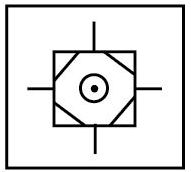Detailed Solution for SSC CGL Tier 1 (19 October) Shift 1 Past Year 2014 Paper - Question 40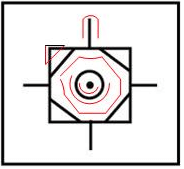From above we can get parts as-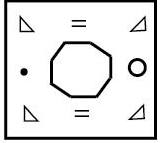Hence, Option B is correct.

SSC CGL Tier 1 (19 October) Shift 1 Past Year 2014 Paper - Question 41

Which of the following answer figures cannot occur when the question figure given below is rotated ?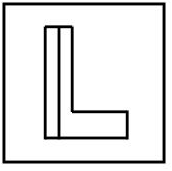Detailed Solution for SSC CGL Tier 1 (19 October) Shift 1 Past Year 2014 Paper - Question 41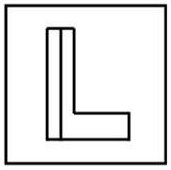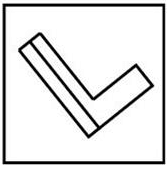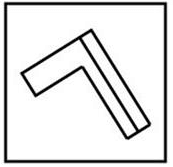Option A,B,D can be made by rotating the figure in anti-clockwise direction.
Option C is formed by flipping the figure and then rotating.
Hence, Option C is the correct answer.

SSC CGL Tier 1 (19 October) Shift 1 Past Year 2014 Paper - Question 42

In each of the following questions, which of the following Venn diagrams best represents relation between given classes ?

Humans, Birds, Animals

Detailed Solution for SSC CGL Tier 1 (19 October) Shift 1 Past Year 2014 Paper - Question 42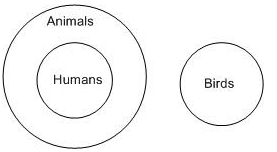Since, all humans are animals but birds belong to different class,
Therefore, Option C is correct

SSC CGL Tier 1 (19 October) Shift 1 Past Year 2014 Paper - Question 43

In each of the following questions, which of the following Venn diagrams best represents relation between given classes ?

Fruits, Apples, Oranges

Detailed Solution for SSC CGL Tier 1 (19 October) Shift 1 Past Year 2014 Paper - Question 43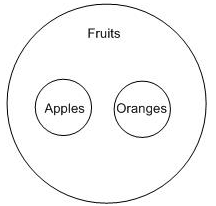Since, Apples and Oranges are both fruits.
Hence, Options B is correct.

SSC CGL Tier 1 (19 October) Shift 1 Past Year 2014 Paper - Question 44

In each of the following questions, which of the following Venn diagrams best represents relation between given classes ?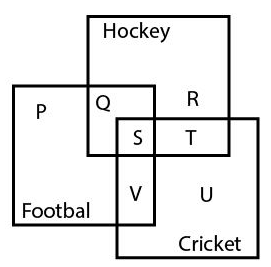Detailed Solution for SSC CGL Tier 1 (19 October) Shift 1 Past Year 2014 Paper - Question 44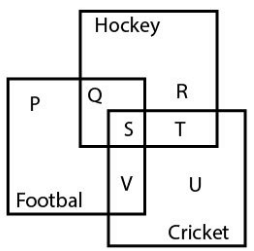According to the figure,
Person, who plays cricket as well as Hockey and Football has to be the one which is common to all 3 categories in the figure.
From the figure, it is clear that 'S' is the only such person.
Hence,Option C is correct.

SSC CGL Tier 1 (19 October) Shift 1 Past Year 2014 Paper - Question 45

In each of the following questions, which answer figure will complete the pattern in the question figure?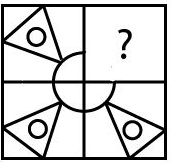Detailed Solution for SSC CGL Tier 1 (19 October) Shift 1 Past Year 2014 Paper - Question 45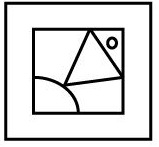fits the figure.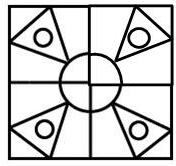Hence, Option A is correct.

SSC CGL Tier 1 (19 October) Shift 1 Past Year 2014 Paper - Question 46

In each of the following questions, which answer figure will complete the pattern in the question figure?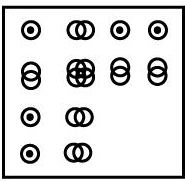Detailed Solution for SSC CGL Tier 1 (19 October) Shift 1 Past Year 2014 Paper - Question 46

Question figure has series of circle which gets merge in second row and second column.
Hence the figure that will complete the given image must have 4 circles which is option A.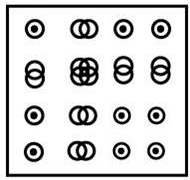Hence, Option A is correct.

SSC CGL Tier 1 (19 October) Shift 1 Past Year 2014 Paper - Question 47

From the given answer figures, select the one in which the question figure is hidden/embedded.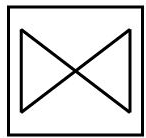Detailed Solution for SSC CGL Tier 1 (19 October) Shift 1 Past Year 2014 Paper - Question 47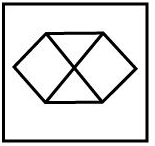Remove side edges from above figures.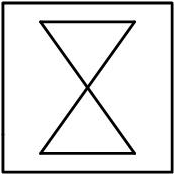Rotate above figure by 90 degree.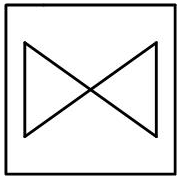Hence, Option A is correct.

SSC CGL Tier 1 (19 October) Shift 1 Past Year 2014 Paper - Question 48

A piece of paper is folded and cut as shown below in th question figures. From the given answer figures, indicate how it will appear when opened.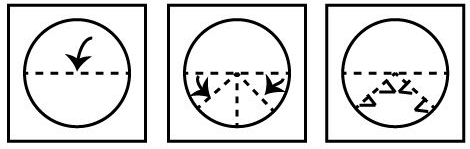Detailed Solution for SSC CGL Tier 1 (19 October) Shift 1 Past Year 2014 Paper - Question 48

By paper folding and cutting according to questions,we get figure as-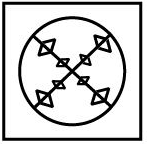Hence, Option C is correct.

SSC CGL Tier 1 (19 October) Shift 1 Past Year 2014 Paper - Question 49

If a mirror is placed on the line MN, then which of the answer figures is the right image of the given figure ?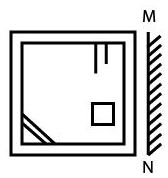Detailed Solution for SSC CGL Tier 1 (19 October) Shift 1 Past Year 2014 Paper - Question 49

A mirror image in a plane mirror is a reflection of an object that appears almost identical but is reversed in the direction perpendicular to the mirror surface.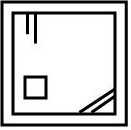Option C fits as the mirror image perfectly.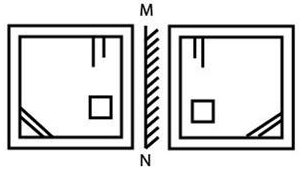SSC CGL Tier 1 (19 October) Shift 1 Past Year 2014 Paper - Question 50

A word is represented by only one set of numbers as given in any one of the alternatives. The sets of numbers given in the alternatives are represented by two classes of alphabets as in two matrices given below. The columns and rows of Matrix I are numbered from 0 to 4 and 2 to 6 respectively and that of Matrix II are numbered from 2 to 6 and 7 to 0 respectively. A letter from these matrices can be represented first by its row and next by its column, e.g., ‘H’ can be represented by 04, 25, 32, etc., and ‘N’ can be represented by 21, 40, 59, etc. Similarly, you have to identify the set for the word given below YEAR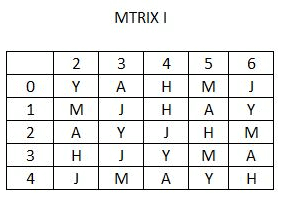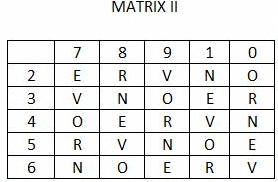Detailed